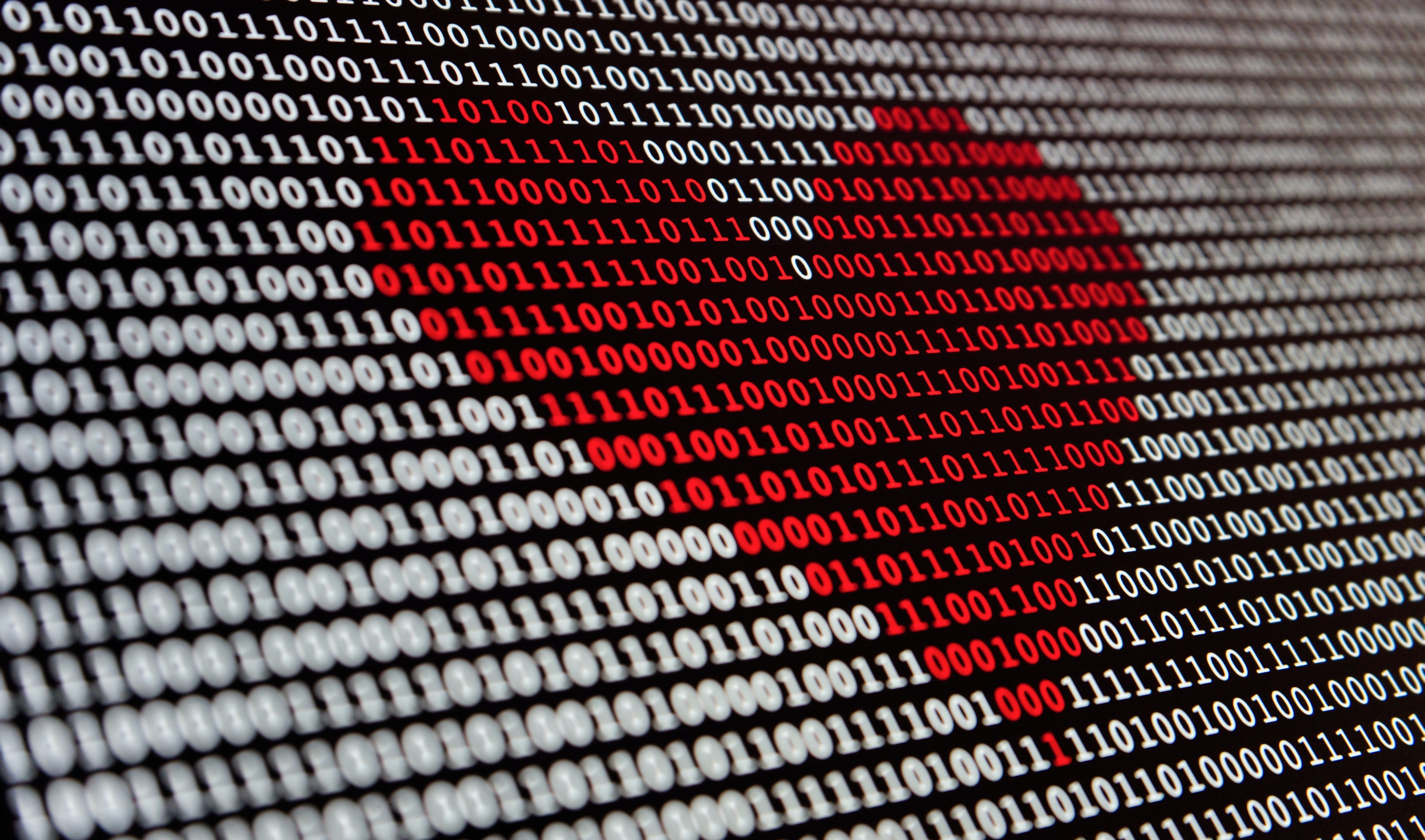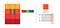# LSB Image Steganography Using PythonPhoto by Alexander Sinn on Unsplash

Hello there!

In this article, we will understand how to implement Least-Significant Bit Steganography using Python.

# WHAT IS STEGANOGRAPHY?

Steganography is the science that involves communicating secret data in an appropriate multimedia carrier, e.g., image, audio, and video files. It comes under the assumption that if the feature is visible, the point of attack is evident, thus the goal here is always to conceal the very existence of the embedded data.

# LSB IMAGE STEGANOGRAPHY

LSB Steganography is an image steganography technique in which messages are hidden inside an image by replacing each pixel’s least significant bit with the bits of the message to be hidden.

To understand better, let’s consider a digital image to be a 2D array of pixels. Each pixel contains values depending on its type and depth. We will consider the most widely used modes — RGB(3x8-bit pixels, true-color) and RGBA(4x8-bit pixels, true-color with transparency mask). These values range from 0–255, (8-bit values).Representation of Image as a 2D Array of RGB Pixels

We can convert the message into decimal values and then into binary, by using the ASCII Table. Then, we iterate over the pixel values one by one, after converting them to binary, we replace each least significant bit with that message bits in a sequence.

To decode an encoded image, we simply reverse the process. Collect and store the last bits of each pixel then split them into groups of 8 and convert it back to ASCII characters to get the hidden message.

# PYTHON IMPLEMENTATION

Now, we will try to implement the above concept step-by-step with the help of Python Libraries — PIL and NumPy.

Step 1: Import all the required python libraries

`import numpy as npfrom PIL import Image`

Step 2: Make the Encoder Function

Firstly, we write the code to convert the source image into a NumPy array of pixels and store the size of the image. We check if the mode of the image is RGB or RGBA and consequently set the value of n. We also calculate the total number of pixels.

`def Encode(src, message, dest):    img = Image.open(src, 'r')    width, height = img.size    array = np.array(list(img.getdata()))    if img.mode == 'RGB':        n = 3    elif img.mode == 'RGBA':        n = 4    total_pixels = array.size//n`

Secondly, we add a delimiter (“\$t3g0") at the end of the secret message, so that when the program decodes, it knows when to stop. We convert this updated message to binary form and calculate the required pixels.

`message += "\$t3g0"b_message = ''.join([format(ord(i), "08b") for i in message])req_pixels = len(b_message)`

Thirdly, we make a check if the total pixels available is sufficient for the secret message or not. If yes, we proceed to iterating the pixels one by one and modifying their least significant bits to the bits of the secret message until the complete message including the delimiter has been hidden.

`if req_pixels > total_pixels:    print("ERROR: Need larger file size")else:    index=0    for p in range(total_pixels):        for q in range(0, 3):            if index < req_pixels:                array[p][q] = int(bin(array[p][q])[2:9] + b_message[index], 2)                index += 1`

Finally, we have the updated pixels array and we can use this to create and save it as the destination output image.

`array=array.reshape(height, width, n)enc_img = Image.fromarray(array.astype('uint8'), img.mode)enc_img.save(dest)print("Image Encoded Successfully")`

With this, our encoder function is done and should look something like this —

`def Encode(src, message, dest):    img = Image.open(src, 'r')    width, height = img.size    array = np.array(list(img.getdata()))    if img.mode == 'RGB':        n = 3    elif img.mode == 'RGBA':        n = 4    total_pixels = array.size//n    message += "\$t3g0"    b_message = ''.join([format(ord(i), "08b") for i in message])    req_pixels = len(b_message)    if req_pixels > total_pixels:        print("ERROR: Need larger file size")    else:        index=0        for p in range(total_pixels):            for q in range(0, 3):                if index < req_pixels:                    array[p][q] = int(bin(array[p][q])[2:9] + b_message[index], 2)                    index += 1        array=array.reshape(height, width, n)        enc_img = Image.fromarray(array.astype('uint8'), img.mode)        enc_img.save(dest)        print("Image Encoded Successfully")`

Step 3: Make the Decoder Function

Firstly, we repeat a similar procedure of saving the pixels of the source image as an array, figuring out the mode, and calculating the total pixels.

`def Decode(src):    img = Image.open(src, 'r')    array = np.array(list(img.getdata()))    if img.mode == 'RGB':        n = 3    elif img.mode == 'RGBA':        n = 4    total_pixels = array.size//n`

Secondly, we need to extract the least significant bits from each of the pixels starting from the top-left of the image and store it in groups of 8. Next, we convert these groups into ASCII characters to find the hidden message until we read the delimiter inserted previously completely.

`hidden_bits = ""for p in range(total_pixels):    for q in range(0, 3):        hidden_bits += (bin(array[p][q])[2:][-1])hidden_bits = [hidden_bits[i:i+8] for i in range(0, len(hidden_bits), 8)]message = ""for i in range(len(hidden_bits)):    if message[-5:] == "\$t3g0":        break    else:        message += chr(int(hidden_bits[i], 2))`

Finally, we do a check if the delimiter was found or not. If not, that means there was no hidden message in the image.

`if "\$t3g0" in message:    print("Hidden Message:", message[:-5])else:    print("No Hidden Message Found")`

With this, our decoder function is done and should look something like this —

`def Decode(src):    img = Image.open(src, 'r')    array = np.array(list(img.getdata()))    if img.mode == 'RGB':        n = 3    elif img.mode == 'RGBA':        n = 4    total_pixels = array.size//n    hidden_bits = ""    for p in range(total_pixels):        for q in range(0, 3):            hidden_bits += (bin(array[p][q])[2:][-1])    hidden_bits = [hidden_bits[i:i+8] for i in range(0, len(hidden_bits), 8)]    message = ""    for i in range(len(hidden_bits)):        if message[-5:] == "\$t3g0":            break        else:            message += chr(int(hidden_bits[i], 2))    if "\$t3g0" in message:        print("Hidden Message:", message[:-5])    else:        print("No Hidden Message Found")`

Step 4: Make the Main Function

For the main function, we ask the user which function they would like to perform — Encode or Decode.

For Encode, we ask the user the following inputs — source image name with extension, secret message, and destination image name with extension.

For Decode, we ask the user for the source image, that has a message hidden.

`def Stego():    print("--Welcome to \$t3g0--")    print("1: Encode")    print("2: Decode")    func = input()    if func == '1':        print("Enter Source Image Path")        src = input()        print("Enter Message to Hide")        message = input()        print("Enter Destination Image Path")        dest = input()        print("Encoding...")        Encode(src, message, dest)    elif func == '2':        print("Enter Source Image Path")        src = input()        print("Decoding...")        Decode(src)    else:        print("ERROR: Invalid option chosen")`

Step 5: Put all the above functions together and our own LSB Image Steganography program is ready. Check out my GitHub repository for the complete code.

NOTE

In a study, it was observed that conventional LSB is not effective in the case of JPEG as the data gets manipulated on compression due to its lossy nature. Whereas for a PNG image a simple LSB is applicable without any loss of data on compression. So, try running your program on PNG images only.

# EXAMPLE

1. Encode Message

2. Decode Message

I extensively use this Online Steganography Tool in CTFs, was intrigued to know the code behind its functioning and that’s all the story behind approaching this advanced topic for today.

Thank you for reading this article, hope you got to learn something.

Catch you in the next one!

Support me https://www.buymeacoffee.com/djrobin17

# REFERENCES

## The Startup

Get smarter at building your thing. Join The Startup’s +799K followers.

Written by

## Devang Jain

CyberSecurity Enthusiast | Filmmaker & Designer | CSE Undergraduate

## The Startup

Get smarter at building your thing. Follow to join The Startup’s +8 million monthly readers & +799K followers.

Written by

## Devang Jain

CyberSecurity Enthusiast | Filmmaker & Designer | CSE Undergraduate## The Startup

Get smarter at building your thing. Follow to join The Startup’s +8 million monthly readers & +799K followers.

## More From Medium

Medium is an open platform where 170 million readers come to find insightful and dynamic thinking. Here, expert and undiscovered voices alike dive into the heart of any topic and bring new ideas to the surface. Learn more

Follow the writers, publications, and topics that matter to you, and you’ll see them on your homepage and in your inbox. Explore

If you have a story to tell, knowledge to share, or a perspective to offer — welcome home. It’s easy and free to post your thinking on any topic. Write on Medium